# IndexError: tuple index out of range in Python [Solved]Last updated: Feb 16, 2023
5 min## #IndexError: tuple index out of range in Python

The Python "IndexError: tuple index out of range" occurs when we try to access an index that doesn't exist in a tuple.

Indexes are zero-based in Python, so the index of the first item in the tuple is `0`, and the index of the last is `-1` or `len(my_tuple) - 1`.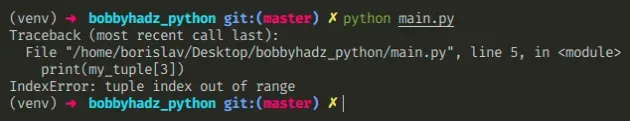Here is an example of how the error occurs.

main.py
```Copied!```my_tuple = ('a', 'b', 'c')

# ⛔️ IndexError: tuple index out of range
print(my_tuple)
``````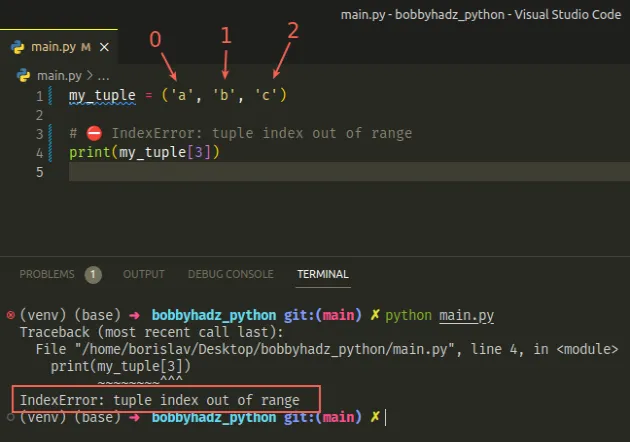The tuple has a length of `3`. Since indexes in Python are zero-based, the first item in the tuple has an index of `0`, and the last an index of `2`.

abc
012
If we try to access any positive index outside the range of `0-2`, we would get an `IndexError`.

## #Getting the last item of a tuple

If you need to get the last item in a tuple, use `-1`.

main.py
```Copied!```my_tuple = ('a', 'b', 'c')

print(my_tuple[-1])  # 👉️ c
print(my_tuple[-2])  # 👉️ b
``````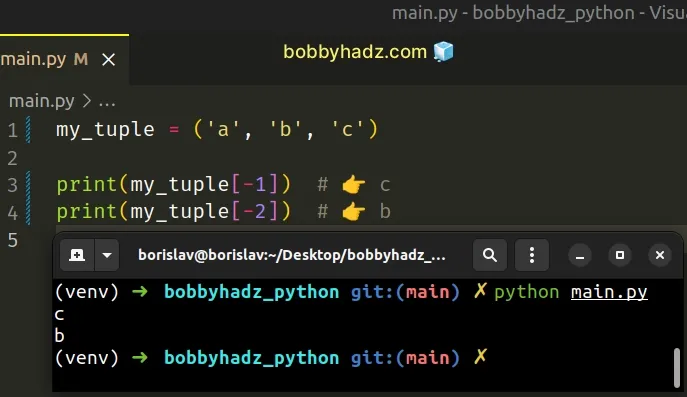When the index starts with a minus, we start counting backward from the end of the tuple.

## #Getting the length of a tuple

If you need to get the length of the tuple, use the `len()` function.

main.py
```Copied!```my_tuple = ('a', 'b', 'c')

print(len(my_tuple)) # 👉️ 3
``````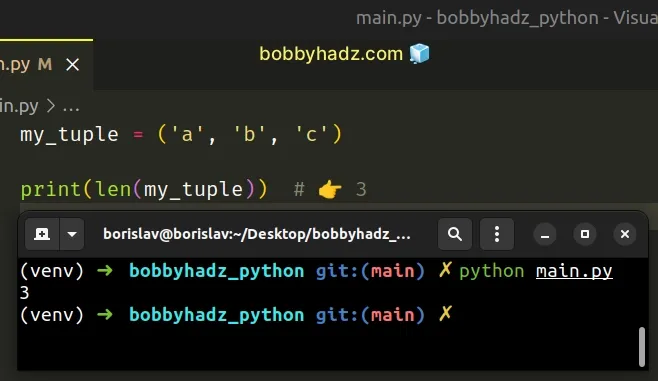The len() function returns the length (the number of items) of an object.

The argument the function takes may be a sequence (a string, tuple, list, range or bytes) or a collection (a dictionary, set, or frozen set).

If you need to check if an index exists before accessing it, use an `if` statement.

main.py
```Copied!```my_tuple = ('a', 'b', 'c')

idx = 3

if len(my_tuple) > idx:
print(my_tuple[idx])
else:
# 👇️ this runs
print(f'index {idx} is out of range')
``````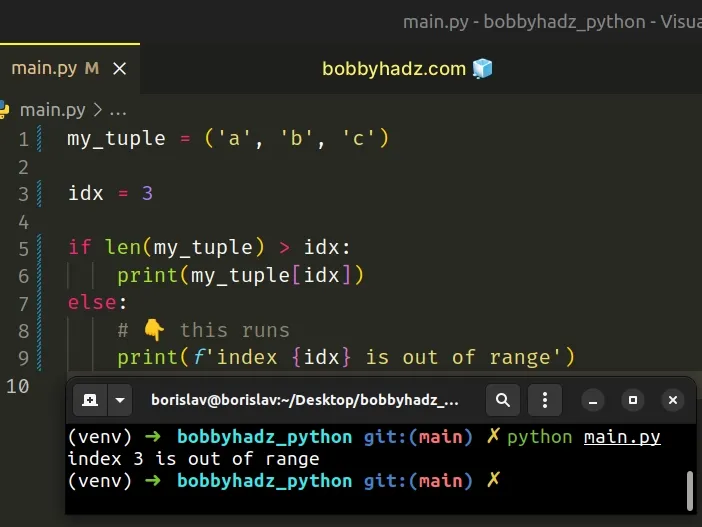If a tuple has a length of `3`, then its last index is `2` (because indexes are zero-based).

This means that you can check if the tuple's length is greater than the index you are trying to access.

## #Iterating over a tuple with range()

If you are trying to iterate over a tuple using the `range()` class, use the length of the tuple.

main.py
```Copied!```my_tuple = ('a', 'b', 'c')

for i in range(len(my_tuple)):
print(i, my_tuple[i])  # 👉️ 0 a, 1 b, 2 c
``````However, a much better solution is to use the `enumerate()` function to iterate over a tuple with access to the index.

main.py
```Copied!```my_tuple = ('a', 'b', 'c')

for index, item in enumerate(my_tuple):
print(index, item)  # 👉️ 0 a, 1 b, 2 c
``````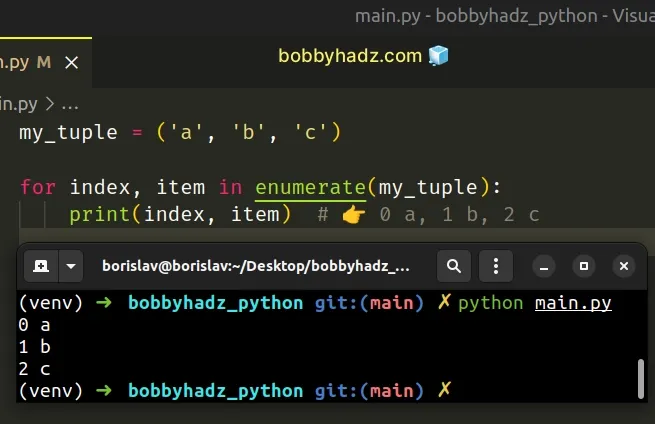The enumerate function takes an iterable and returns an enumerate object containing tuples where the first element is the index, and the second is the item.

## #Using a `try/except` statement to handle the error

An alternative approach to handle the exception is to use a try/except block.

main.py
```Copied!```my_tuple = ('a', 'b', 'c')

try:
result = my_tuple
except IndexError:
# 👇️ this runs
print('index out of range')
``````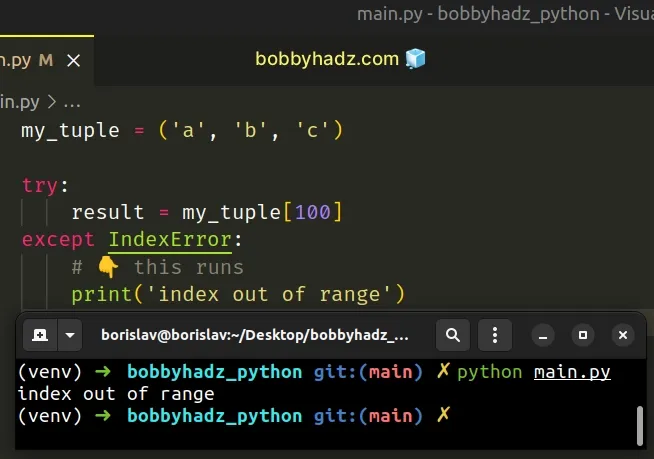We tried accessing the tuple item at index `100` which raised an `IndexError` exception.

You can handle the error or use the `pass` keyword in the `except` block.

main.py
```Copied!```my_tuple = ('a', 'b', 'c')

try:
result = my_tuple
except IndexError:
pass
``````

The pass statement does nothing and is used when a statement is required syntactically but the program requires no action.

## #Trying to access an empty tuple at any index causes the error

Note that if you try to access an empty tuple at a specific index, you'd always get an `IndexError`.

main.py
```Copied!```my_tuple = ()

print(my_tuple) # 👉️ ()
print(len(my_tuple)) # 👉️ 0

# ⛔️ IndexError: tuple index out of range
print(my_tuple)
``````

You should print the tuple you are trying to access and its length to make sure the variable stores what you expect.

Tuples are very similar to lists, but implement fewer built-in methods and are immutable (cannot be changed).

In case you declared a tuple by mistake, tuples are constructed in multiple ways:

• Using a pair of parentheses `()` creates an empty tuple
• Using a trailing comma - `a,` or `(a,)`
• Separating items with commas - `a, b` or `(a, b)`
• Using the `tuple()` constructor

## #The error isn't raised when using tuple slicing

Note that the error isn't raised when using tuple slicing.

The syntax for tuple slicing is `my_tuple[start:stop:step]`.

The `start` index is inclusive and the `stop` index is exclusive (up to, but not including).
main.py
```Copied!```my_tuple = ('a', 'b', 'c', 'd')

print(my_tuple[0:2])  # 👉️ ('a', 'b')
print(my_tuple[2:100])  # 👉️ ('c', 'd')
``````

The slice `my_tuple[0:2]` starts at index `0` and goes up to, but not including index `2`.

The slice `my_tuple[2:100]` starts at index `2` and goes up to, but not including index `100`.

If the `start` index is omitted, it is considered to be `0` and if the `stop` index is omitted, the slice goes to the end of the tuple.

main.py
```Copied!```print(my_tuple[2:])  # 👉️ ('c', 'd')
print(my_tuple[:2])  # 👉️ ('a', 'b')
``````

The slice `my_tuple[2:]` starts at index `2` and goes to the end of the tuple.

The slice `my_tuple[:2]` starts at index `0` and goes up to, but not including index `2`.

Python indexes are zero-based, so the first item in a tuple has an index of `0`, and the last item has an index of `-1` or `len(my_tuple) - 1`.

## #Use a `try/except` statement if taking the index from user input

If you are taking an index from user input, use a `try/except` statement to handle the error if the user enters an index that is out of range.

main.py
```Copied!```my_tuple = ('a', 'b', 'c', 'd')

index = int(input('Enter an index: '))

try:
value = my_tuple[index]
print(f'✅ The value is: {value}')
except IndexError:
print('⛔️ The specified index does NOT exist')
``````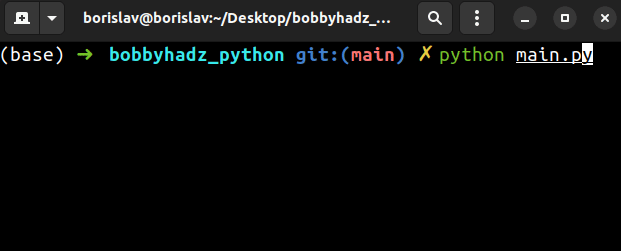The tuple has `4` elements, so the last item has an index of `3` because indices are zero-based.

If the user enters an index that is greater than `3`, an `IndexError` is raised an the `except` block runs.

Otherwise, we access the tuple at the specified index and print the value.